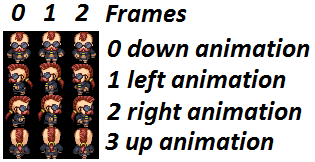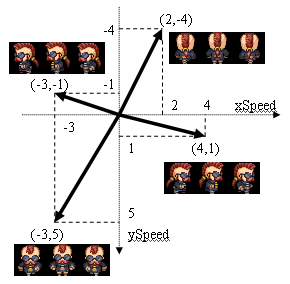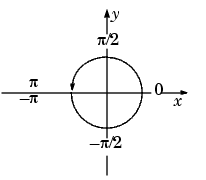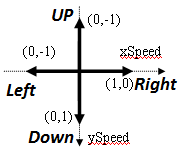# Sprites: Speed and animation. Android Game Programming 5.

In this tutorial we are going to give to our sprite a random x and y speed. With this, the sprite will start with an aleatory direction that is going to change each time it reaches a border.

package com.edu4java.android.killthemall;

import java.util.Random;

import android.graphics.Bitmap;

import android.graphics.Canvas;

import android.graphics.Rect;

public class Sprite {

private static final int BMP_ROWS = 4;

private static final int BMP_COLUMNS = 3;

private int x = 0;

private int y = 0;

private int xSpeed = 5;

private GameView gameView;

private Bitmap bmp;

private int currentFrame = 0;

private int width;

private int height;

private int ySpeed;

public Sprite(GameView gameView, Bitmap bmp) {

this.gameView = gameView;

this.bmp = bmp;

this.width = bmp.getWidth() / BMP_COLUMNS;

this.height = bmp.getHeight() / BMP_ROWS;

Random rnd = new Random();

xSpeed = rnd.nextInt(10)-5;

ySpeed = rnd.nextInt(10)-5;

}

private void update() {

if (x > gameView.getWidth() - width - xSpeed || x + xSpeed < 0) {

xSpeed = -xSpeed;

}

x = x + xSpeed;

if (y > gameView.getHeight() - height - ySpeed || y + ySpeed < 0) {

ySpeed = -ySpeed;

}

y = y + ySpeed;

currentFrame = ++currentFrame % BMP_COLUMNS;

}

public void onDraw(Canvas canvas) {

update();

int srcX = currentFrame * width;

int srcY = 1 * height;

Rect src = new Rect(srcX, srcY, srcX + width, srcY + height);

Rect dst = new Rect(x, y, x + width, y + height);

canvas.drawBitmap(bmp, src, dst, null);

}

}

Our Sprite image contains 4 animations with 3 frames each:Depending on which direction the sprite is going we have to show a different animation, for example:We need a function that gets as parameters xSpeed and ySpeed and returns the row we have to use for the animation [0,1,2,3]

We are going to use the Math.atan2(xSpeed, ySpeed) function to calculate the Sprite direction in execution time.

atan2(x,y) gives the radian angle in double from (-PI to PI) but we just want an int result from 0 to 3 to know which animation we have to use.Lets consider the next graphic, I write the coordinates (x,y) for each direction that we have an animation: Up (0,-1), right (1,0), down (0,1) and left (0,-1).When the result angle is near to one of this direction we want to use the corresponding up, right, down or left animation. To do that I use Math.round function.

Ok, now the hard part; I solved this with this function and one constant array:

// direction = 0 up, 1 left, 2 down, 3 right,

// animation = 3 up, 1 left, 0 down, 2 right

int[] DIRECTION_TO_ANIMATION_MAP = { 3, 1, 0, 2 };

private int getAnimationRow() {

double dirDouble = (Math.atan2(xSpeed, ySpeed) / (Math.PI / 2) + 2);

int direction = (int) Math.round(dirDouble) % BMP_ROWS;

return DIRECTION_TO_ANIMATION_MAP[direction];

}

In this table I try to show you what I do in this function:

1. with atan2(xSpeed,ySpeed) I get the radian angle from (-PI to PI)
2. I divide the angle by PI/2 and get a double from (-2 to 2)
3. I add 2 to change the range from (0 to 4)
4. I use % (module) to reduce the range to (0 to 3) (notice the 0 and 4 were the same direction)
5. I map each direction to the correct animation using the array { 3, 1, 0, 2 }
 x y atan2(x,y) atan2(x,y)/(PI/2) (atan2(x,y)/(PI/2)+2)%4 bmp row (from 0) up 0 -1 PI or -PI 2 or -2 4 or 0 3 right 1 0 PI/2 1 3 2 down 0 1 0 0 2 0 left -1 0 -PI/2 -1 1 1

Note: here x and y are xSpeed and ySpeed.

If you are still reading, congratulations, you are a Game Programmer ;)

package com.edu4java.android.killthemall;

import java.util.Random;

import android.graphics.Bitmap;

import android.graphics.Canvas;

import android.graphics.Rect;

public class Sprite {

// direction = 0 up, 1 left, 2 down, 3 right,

// animation = 3 back, 1 left, 0 front, 2 right

int[] DIRECTION_TO_ANIMATION_MAP = { 3, 1, 0, 2 };

private static final int BMP_ROWS = 4;

private static final int BMP_COLUMNS = 3;

private int x = 0;

private int y = 0;

private int xSpeed = 5;

private GameView gameView;

private Bitmap bmp;

private int currentFrame = 0;

private int width;

private int height;

private int ySpeed;

public Sprite(GameView gameView, Bitmap bmp) {

this.width = bmp.getWidth() / BMP_COLUMNS;

this.height = bmp.getHeight() / BMP_ROWS;

this.gameView = gameView;

this.bmp = bmp;

Random rnd = new Random(System.currentTimeMillis());

xSpeed = rnd.nextInt(50) - 5;

ySpeed = rnd.nextInt(50) - 5;

}

private void update() {

if (x >= gameView.getWidth() - width - xSpeed || x + xSpeed <= 0) {

xSpeed = -xSpeed;

}

x = x + xSpeed;

if (y >= gameView.getHeight() - height - ySpeed || y + ySpeed <= 0) {

ySpeed = -ySpeed;

}

y = y + ySpeed;

currentFrame = ++currentFrame % BMP_COLUMNS;

}

public void onDraw(Canvas canvas) {

update();

int srcX = currentFrame * width;

int srcY = getAnimationRow() * height;

Rect src = new Rect(srcX, srcY, srcX + width, srcY + height);

Rect dst = new Rect(x, y, x + width, y + height);

canvas.drawBitmap(bmp, src, dst, null);

}

// direction = 0 up, 1 left, 2 down, 3 right,

// animation = 3 back, 1 left, 0 front, 2 right

private int getAnimationRow() {

double dirDouble = (Math.atan2(xSpeed, ySpeed) / (Math.PI / 2) + 2);

int direction = (int) Math.round(dirDouble) % BMP_ROWS;

return DIRECTION_TO_ANIMATION_MAP[direction];

}

}

 << Our First Sprite Working with Multiple Sprites >>